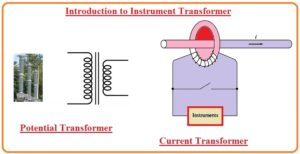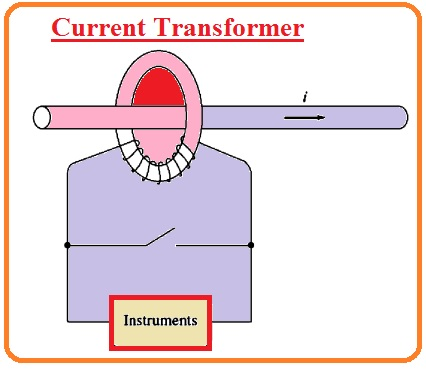Hello, readers I hope you all are enjoying your life. In this tutorial, we will have a detailed look at Introduction to Instrument Transformer. The transformer is such an electrical device that employed in the power system to vary the voltage level from one to other according to requirements of the system and load like to the transformer. According to the structure and uses, there are many types of transformer employed in the system.

An instrument transformer is a transformer type that offers a large value of current and voltage measurements. Their main function is to vary the current and voltage at such a level that can be easily measured through the use of meters like voltmeter and ammeter since the high value of current and voltage is not easy to measure. The primary site of this device is linked to the larger value of current and voltage that we have to measure and other to the meter. In this post we cover its working types, features, and other related parameters. So let’s get started with Introduction to Instrument Transformer.

## Introduction to Instrument Transformer

• There are 2 main categories of the transformer are employed to the system to finds different values. The first one is a potential transformer and the second one is the current transformer used to find the current value.
• The configuration of the potential transformer is such that its higher side is primary and lower voltage side is secondary. Its power rating vlaue is very less.• The main function of this device is to deliver and convert the voltage level to such a value that can be easily measured
• As the main function of a transformer is to transform the voltage to such level that can be easily measured. Therefore it should has a high value of accuracy and not make any serious fact at the value of voltage.
• According to the required application, there should be such type of potential transform is selected that can measure the less error value of voltage.
• Current transformers is such device that used to measure the value of current after making it such value that can find easily.
• The circuit diagram of normal current transformer wounded at the ferromagnetic ring having the one primary passing through the mid of the ring.• The ferromagnetic rings grasp and hold the small value of flux coming from the primary.
• Due to this flux value voltage and current generated in the secondary winding of the transformer.
• Contrary to the other transformer types the mutual flux value in the current transformer is less the leakage flux. since there is less tight coupling the voltage and current ratio
• There is a direct relationship between the current existing at the secondary side and with the primary current and the transformer can offer the correct value of current passing in the system where the transformer is employed.
• There is the ratio between primary and secondary windings is a rating of the transformer. The normal current transformer ratio can has a rating ratio 600/5, 800/5 and 1000/5.
• It is significant to retain the current transformer short circuit during operation because a large value of voltage will rely about the open circuit of the secondary side
• In real the different relays and other types of instruments uses the current through the current transformer having a short interlocking that should be off when relay is removed from the system.
• In absence of this category of interlocking large value of voltage will be existing at the secondary side when the relay is eliminated through it.

So that is a detailed post about instruments transformer here i tried to cover the details about the instrument transformer and its types that are potential and current transformer. If you have any further query ask in the comments.. Thanks for reading have a nice day